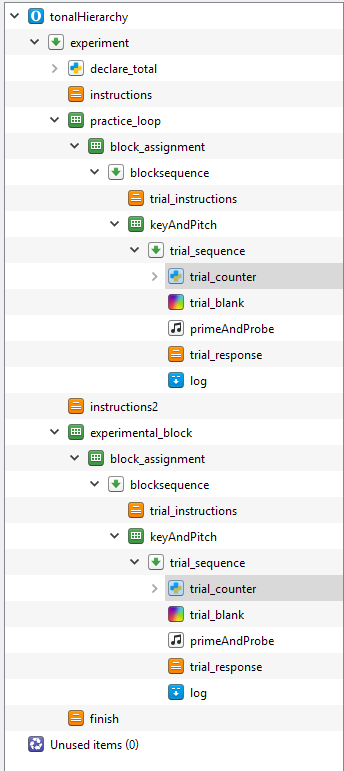Howdy, Stranger!

It looks like you're new here. If you want to get involved, click one of these buttons!

Supported by

trial counter

Hi all,

I'm trying to add a basic trial counter to my experiment ("You are on trial X of Y"). I have read the following threads:

http://forum.cogsci.nl/index.php?p=/discussion/474/solved-how-to-let-the-subject-know-how-many-more-trials-they-have

http://forum.cogsci.nl/index.php?p=/discussion/480/solved-how-to-reset-the-trial-counter-after-the-practice-trials

I have specifically added Lotje's code from the first post to my experiment. Unfortunately, I get the following error when I try to run the experiment:

``````The variable 'count_trial_sequence' does not exist. Tip: Use the variable inspector (Ctrl+I) to see all variables.

item-stack: experiment[run].practice_loop[run].block_assignment[run].blocksequence[run].new_inline_script[run]
time: Mon Jul 16 11:57:07 2018
``````

I've attached a picture of my experiment organization. The file itself is too large to attach, but please let me know if I can upload anything else to make it more clear.• Hi,

I'm not sure whether this will do the trick, but in the loop item, you can check the box `skip on the first iteration` or something like that. If this doesn't help, I would add an inline_script to the block_sequence (the first item or so) and add this line to its prepare phase: `var.count_trial_sequence = 0`

Does that work?

Eudard

• Hi all,

So I've come back to this project after several months. I have been able to implement a counter, and even a counter for my practice trials! Thank you to all for the help!

Unfortunately, I can't seem to figure out how to get the counter to start at 1 rather than 0. I have based my code off of Lotje's code (linked above), but for whatever reason, the following code doesn't seem to do anything on the first iteration of the counter:

`trial_count = trial_count + 1`

I have the following inline script at the very beginning of my experiment (before the first loop):

``````total = 96
practice_total = 5

# To make an in-an-inline_script-item-defined variable
# available in the GUI (e.g., in our case, a sketchpad item)
# as well, we 'set' the variable by using the built-in
# experiment function exp.set().

# This function takes two arguments: the name
# of the variable and its value.
# http://osdoc.cogsci.nl/python-inline-code/experiment-functions/#experiment.set
exp.set("total", total)
exp.set("practice_total", practice_total)
var.trial_count = 0
var.trials_remaining = total
var.practice_trials_remaining = practice_total
``````

And the following inline script in the trial sequence

``````# Determine the trial count.
# The built-in counter 'count_trial_sequence'
# starts counting at zero (in line with the zero-based
# indexing generally used in Python).

# Therefore, we want to add '1' to the
# counter variable you want to show to
# the participant.

# Firstly, we 'get' the in-the-GUI-defined variable
# 'count_trial_sequence' to use it in an inline_script.

# To do so, we use the built-in experiment function
# self.get(). This function takes one argument, namely the
# name of the OpenSesame variable:
# http://osdoc.cogsci.nl/python-inline-code/experiment-functions/#experiment.get

trial_count = self.get("count_trial_sequence")

# Next, we add '1' to the trial_count:
trial_count = trial_count + 1

# To determine how many trials the participant still
# has to do, we use the previously-defined
# variable 'total', which we 'get' with self.get():
trials_remaining = self.get("total") - trial_count

# Finally, we have to 'set' the here-defined variables to
# make them available in the GUI:
exp.set("trial_count", trial_count)
exp.set("trials_remaining", trials_remaining)
``````

Thanks for any help you might be able to provide! I can attach my experiment structure, or any other files that might help answer this question if need be.

• Hi,

In the first part you set `var.trial_count = 0`. However, in the next script, you also have `trial_count = self.get("count_trial_sequence")`. So effectively, you are overwriting the trial count variable every single time with the count_trial_sequence variable. This variable is hardcoded in Opensesame and always starts at 0. I think if you replace `trial_count = self.get("count_trial_sequence")` with `trial_count = self.get("trial_count")`, or simply: `trial_count = var.trial_count`, you should be able to start at 1.

Does that help?

Eduard